文章快速检索 高级检索
 大地测量与地球动力学2022, Vol. 42Issue (1): 35-40  DOI: 10.14075/j.jgg.2022.01.008### 引用本文SUN Wei, ZHU Mingchen. Study on Modeling of Tropospheric Zenith Delay in China with BP-Adaboost Strong Predictor[J]. Journal of Geodesy and Geodynamics, 2022, 42(1): 35-40.### Foundation support

Key Project of College Natural Science Funding of Anhui Provincial Department of Education, No. KJ2018A0480; PhD Candidate Research and Practice Innovation Fund of Jiangsu Province, No. KYCX18_0145; National Undergraduate Training Program for Innovation and Entrepreneurship, No.202110383061.

### Corresponding author

ZHU Mingchen, PhD candidate, lecturer, majors in GNSS meteorology, E-mail: zhumc@seu.edu.cn.

### 第一作者简介

SUN Wei, majors in GNSS meteorology, E-mail: sunweihahahalife@gmail.com.

### 文章历史

1. 铜陵学院建筑工程学院，安徽省铜陵市翠湖四路1335号，244061;
2. 东南大学交通学院，南京市东南大学路2号，218889

1 建模数据与验证数据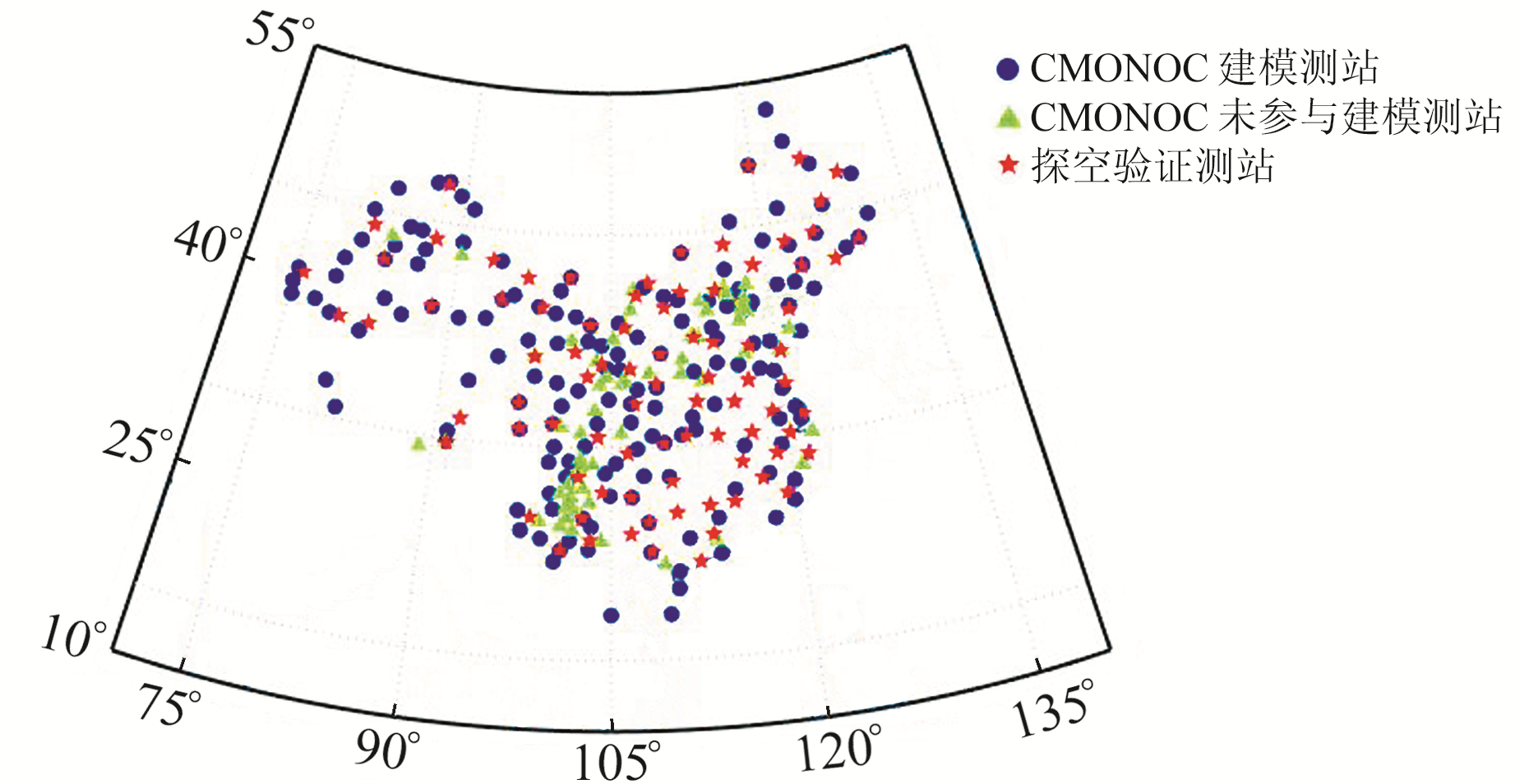图 1 CMONOC与探空测站分布 Fig. 1 Distribution of CMONOC and radiosonde stations

 $\left\{ \begin{array}{l} {\rm{ZHD}} = 0.002\;276{\rm{ }}8 \times \\ \;\;\;\;\frac{P}{{1 - 0.002\;6{\rm{cos}}\left( {2\varphi } \right) - 0.000\;000\;28h}}\\ {\rm{ZWD}} = {10^{ - 6}}\sum\limits_i^{} {} \left[ {\frac{{({h_i}{\rm{ - }}{h_{i + 1}})({N_{{{\rm{w}}_{i + 1}}}}{\rm{ - }}{N_{{{\rm{w}}_i}}})}}{{({\rm{ln}}{N_{{{\rm{w}}_i}}}{\rm{ - ln}}{N_{{{\rm{w}}_{i + 1}}}})}}} \right] \end{array} \right.$ (1)

 ${N_{\rm{w}}} = {\rm{ }}\left( {{k_2}{\rm{ - }}{k_1}\frac{{{R_{\rm{d}}}}}{{{R_{\rm{w}}}}}} \right)\cdot{\rm{ }}\frac{e}{T} + {k_3}\frac{e}{{{T^2}}}$ (2)

 ${h_{\rm{d}}} = {\rm{ }}\frac{C}{{{g_n}}}{\rm{ }} = {\rm{ }}\frac{1}{{{g_n}}}\int_0^h {} g\left( {\varphi , h} \right)\cdot{\rm{d}}h$ (3)
 $\begin{array}{l} g\left( {\varphi , h} \right) = {g_n}(1 - 0.002\;637\;3{\rm{cos}}\left( {2\varphi } \right) + \\ 5.9 \times {10^{ - 6}}{\rm{co}}{{\rm{s}}^2}\left( {2\varphi } \right))\cdot(1 - 3.14 \times {10^{ - 7}}h) \end{array}$ (4)

 $\left\{ \begin{array}{l} {\rm{bias}} = \frac{1}{n}\sum\limits_{i = 1}^n {} ({\rm{ZT}}{{\rm{D}}_i}{\rm{ - ZTD}}_i^0)\\ {\rm{RMS}} = \sqrt {\frac{1}{n}\sum\limits_{i = 1}^n {} {{({\rm{ZT}}{{\rm{D}}_i}{\rm{ - ZTD}}_i^0)}^2}} \end{array} \right.$ (5)

1) 神经网络初始化及训练。弱预测器选取前馈神经网络(feedforward neural network，FNN)。FNN网络由输入层、隐含层、输出层以及模型参数组成，模型的学习误差ε取0.1 mm。FNN网络输入层节点数为5，分别代表测站经度、纬度、高程、年积日(day of year，DOY)和小时；输出层节点数为1，代表ZTD(单位mm)。隐含层节点的选取无规律可循，一般通过试算确定。节点数过多可能导致学习时间过长且结果不稳定，节点数过少会导致网络不收敛。单个FNN网络基本结构如图 2所示。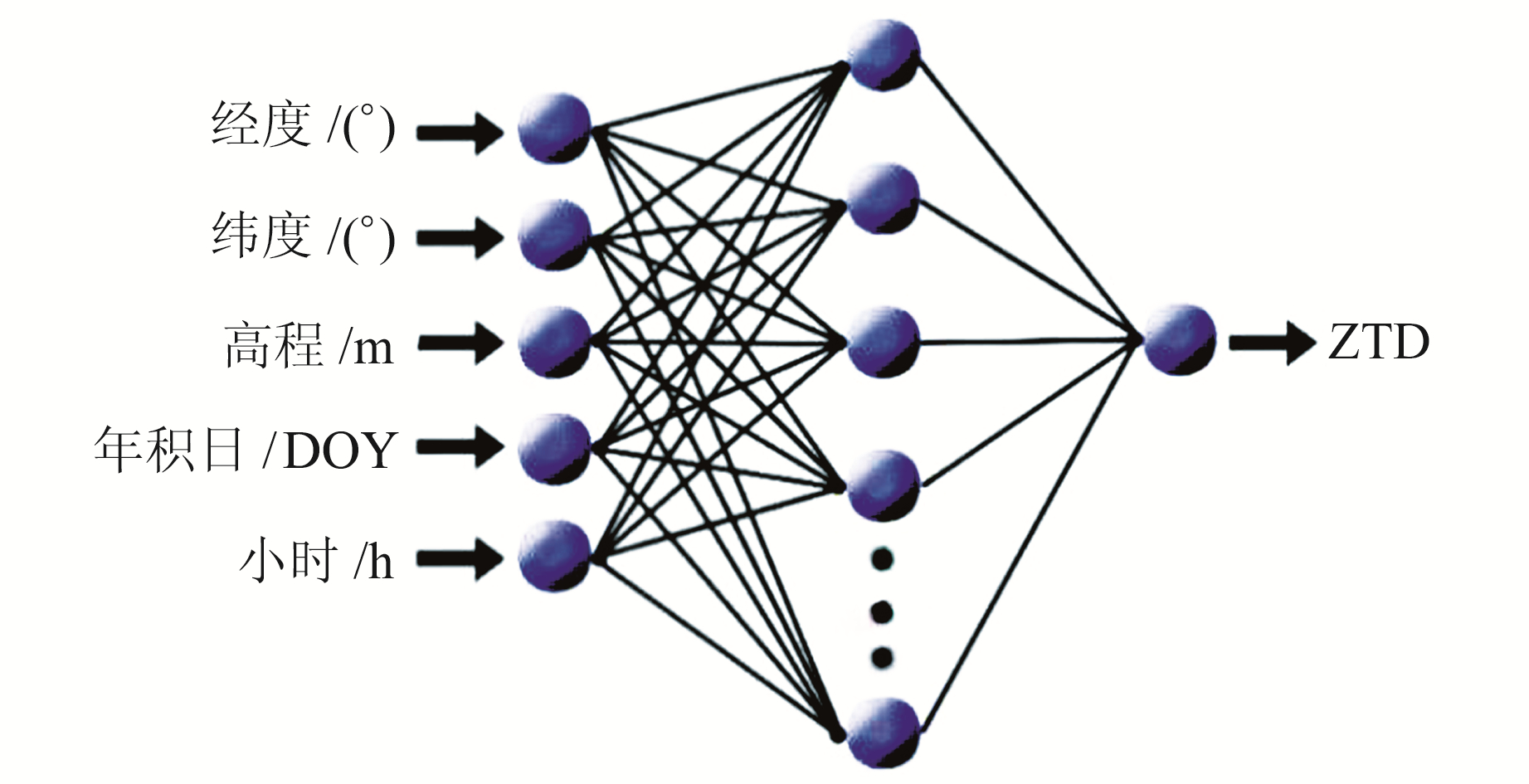图 2 FNN结构 Fig. 2 Feed forward neural network structure

 $f\left( x \right) = {\rm{ }}\frac{2}{{1 + {{\rm{e}}^{{\rm{ - }}2x}}}}{\rm{ }}{\rm{ - }}1$ (6)

FNN训练采用最速下降法，调用MATLAB的toolbox。

2) 累积误差计算。共选取20个FNN网络，将其合并成BP-Adaboost强预测器，用于精确计算ZTD。将n个训练样本的分布权值初始化为D0(i)=1/n, i=1, 2, 3, …, n，每个网络的累积误差初始化为Error(t)=0, t=1, 2, 3, …, 20。若第t个FNN(FNNt)得到的第i个样本的预测误差大于50 mm，则认为该样本是需要加强学习的样本，需要按照公式(7)更新该网络的累积误差Error(t)及样本的分布误差Dt，否则FNNt对应的累积误差及样本的分布权值不变：

 $\begin{array}{l} \left\{ \begin{array}{l} {\rm{Error}}\left( t \right) = {\rm{Error}}\left( t \right) + {D_t}\left( i \right)\\ {D_t}\left( i \right) = {D_{t{\rm{ - }}1}}\left( i \right)\cdot1.1 \end{array} \right.\\ \;\;\;\;\;\;\;\;\;\;t = 1, 2, 3, \ldots , 20 \end{array}$ (7)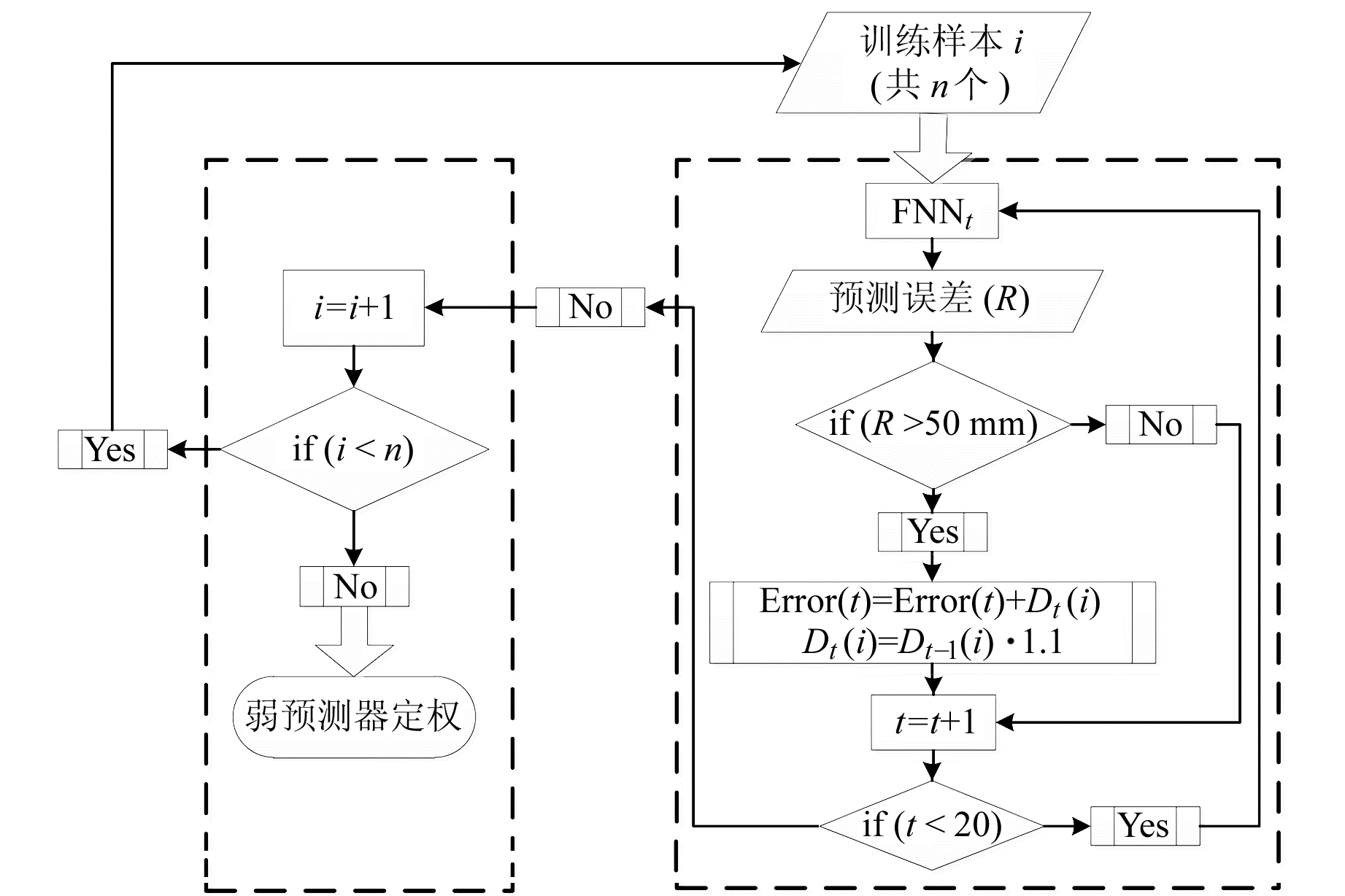图 3 累积误差计算流程 Fig. 3 Cumulative error calculation flow chart

3) 各弱预测器权重计算。第t个FNN网络的权重Pt可由公式(8)计算并归一化得到：

 $\begin{array}{l} \left\{ \begin{array}{l} {p_t} = {\rm{ }}\frac{{0.5}}{{{\rm{exp}}\{ |{\rm{Error}}\left( t \right)|\} }}\\ {p_t} = {\rm{ }}\frac{{{p_t}}}{{\sum\limits_{t = 1}^{20} {} {p_t}}} \end{array} \right.\\ \;\;\;\;\;t = 1, 2, 3, \cdots , 20 \end{array}$ (8)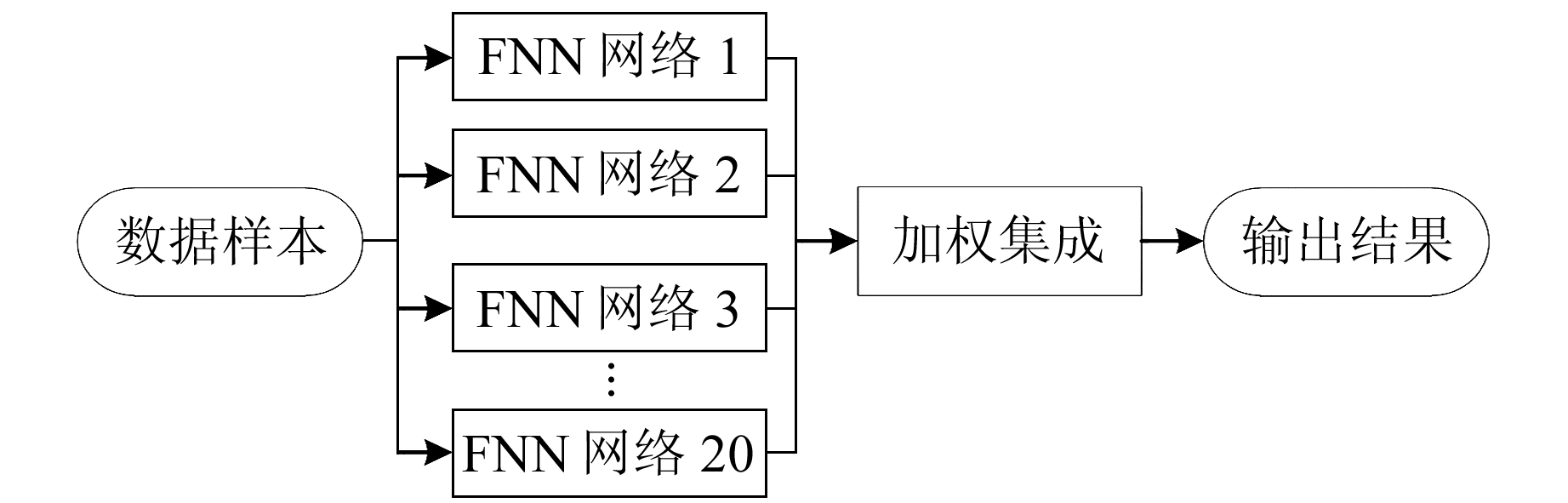图 4 BP-Adaboost算法流程 Fig. 4 The steps of BP-Adaboost algorithm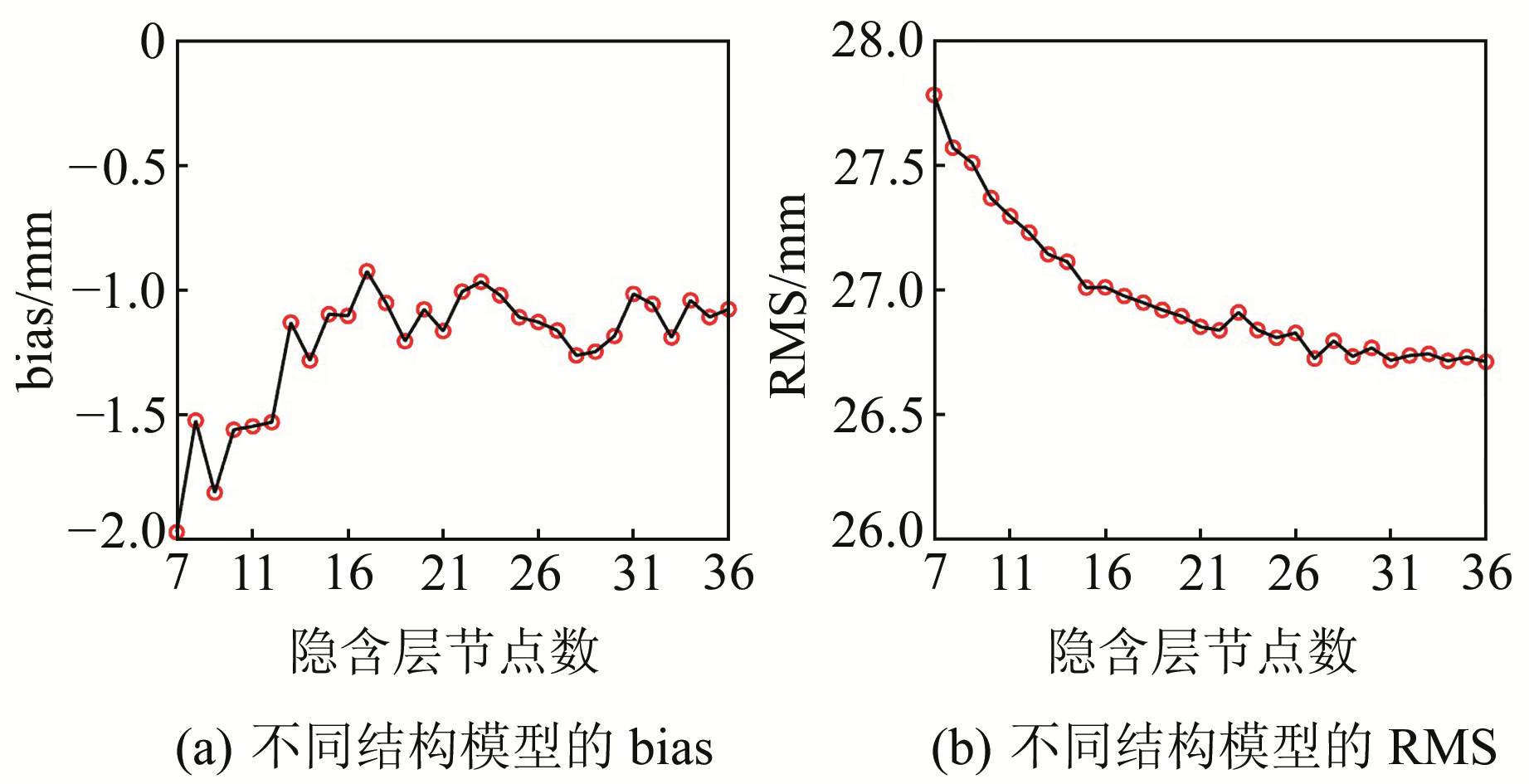图 5 不同隐含层节点数结构的bias和RMS Fig. 5 Bias and RMS with different hidden layer node number structure

3 精度验证表 1 4种模型的bias与RMS Tab. 1 Bias and RMS of the four models

3.1 模型精度的空间分析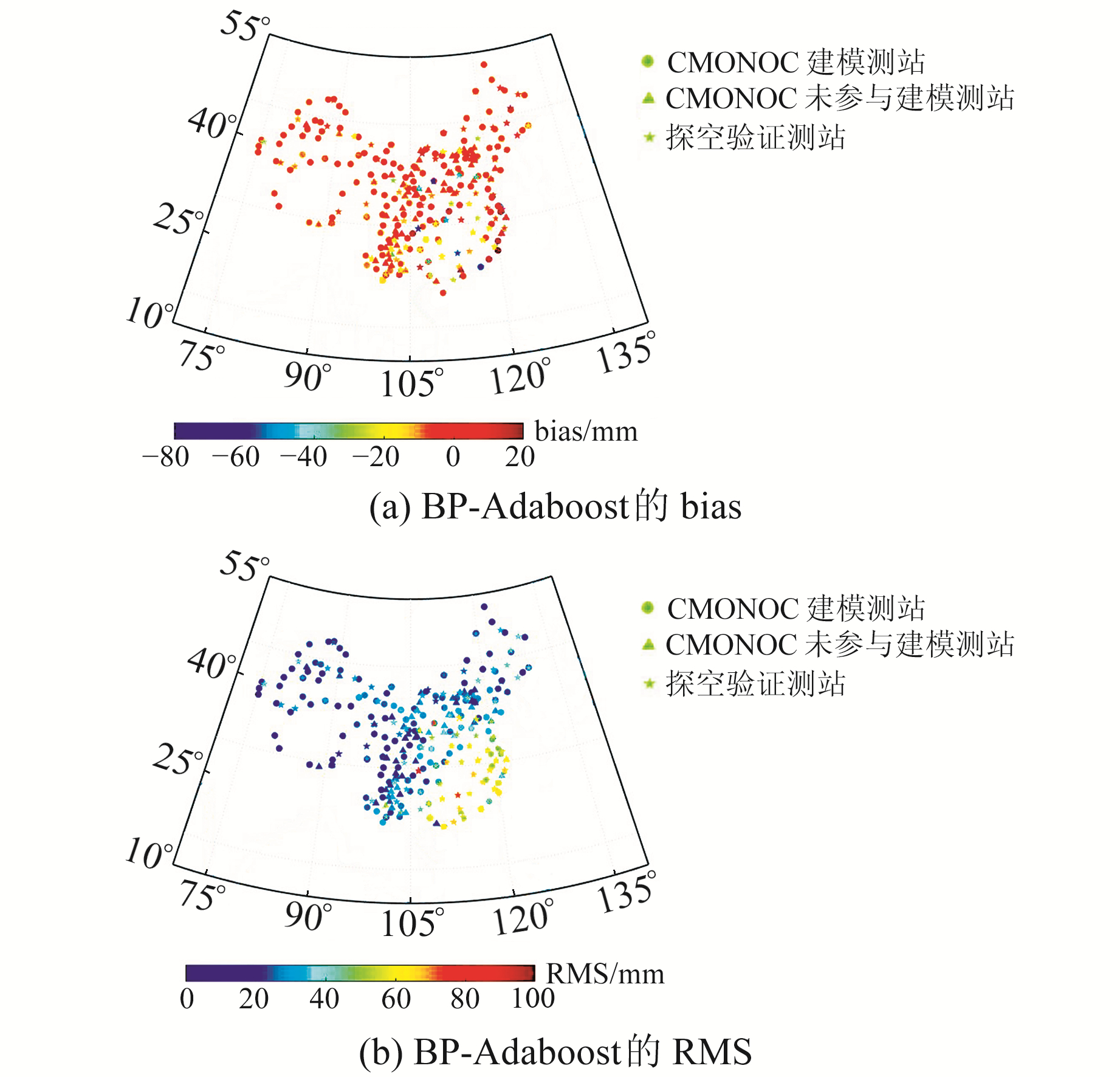图 6 BP-Adaboost模型各测站的bias与RMS分布 Fig. 6 Bias and RMS distribution of each station in BP-Adaboost model表 2 不同纬度区间4种模型的bias与RMS Tab. 2 Bias and RMS of four models in different latitude intervals表 3 3个高程区间的bias和RMS Tab. 3 Bias and RMS of three altitude sections

3.2 模型精度的季节性分析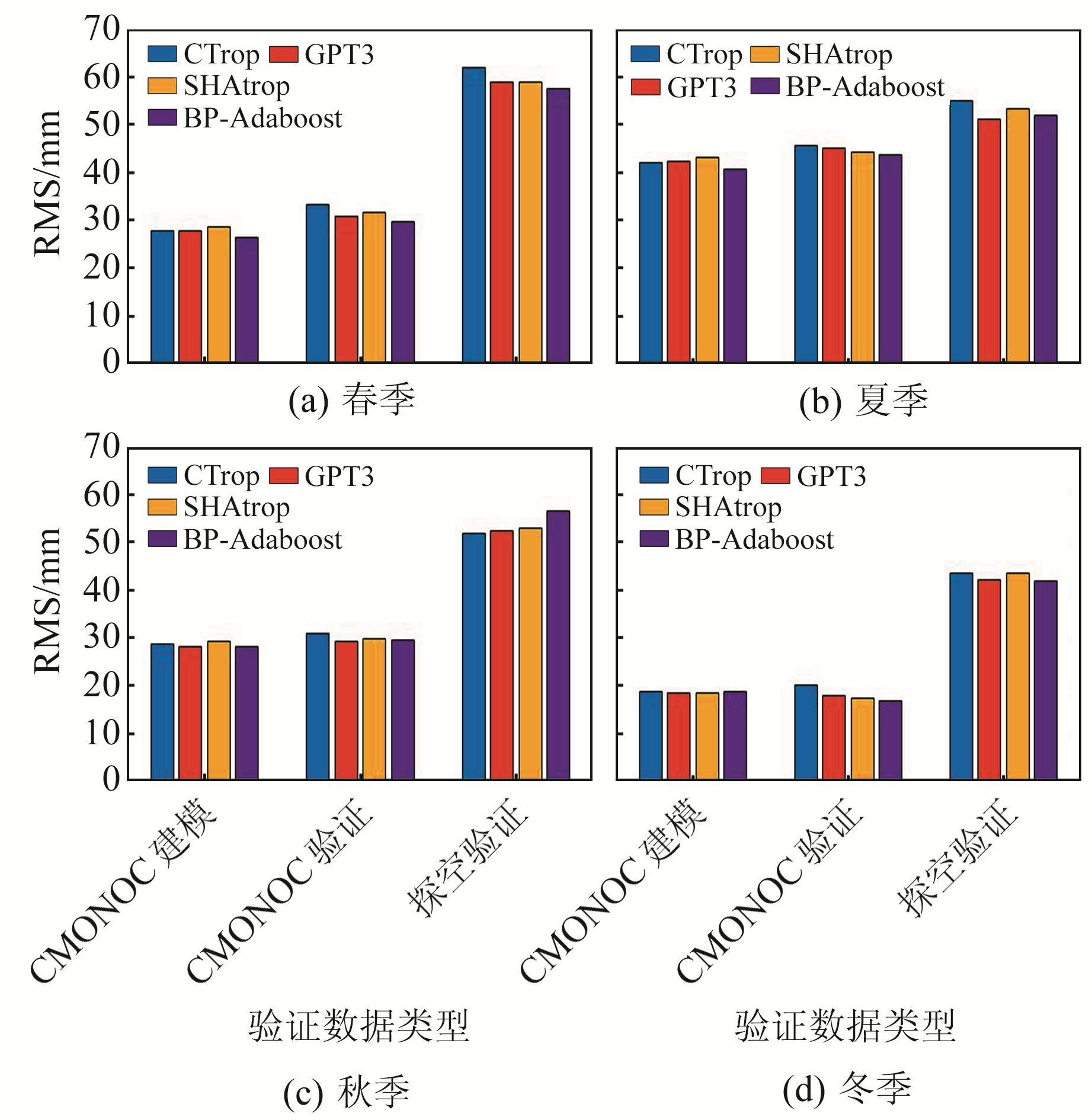图 7 4种模型的季节性RMS Fig. 7 Seasonal RMS of four models

4 结语

1) 利用2019年CMONOC参与建模的155个测站以及未参与建模的62个测站数据进行验证，BP-Adaboost模型在中国区域内的总体bias为0.62 mm和-1.16 mm，优于常用的CTrop模型、GPT3模型和SHAtrop模型，表明该模型没有明显的系统误差；其RMS为25.30 mm和26.72 mm，比上述3种模型精度分别提高9.6%、2.8%和3.3%。

  Hofmann-Wellenhof B, Lichtenegger H, Wasle E. GNSS-Global Navigation Satellite Systems: GPS, GLONASS, Galileo, and More[M]. New York: Springer Science and Business Media, 2007 (0)  Nilsson T, Böhm J, Wijaya D D, et al. Path Delays in the Neutral Atmosphere[M]. Berlin, Heidelberg: Springer Berlin Heidelberg, 2013 (0)  陈俊平, 王君刚, 王解先, 等. SHAtrop: 基于陆态网GNSS数据的中国大陆区域ZTD模型[J]. 武汉大学学报: 信息科学版, 2019, 44(11): 1 588-1 595 (Chen Junping, Wang Jungang, Wang Jiexian, et al. SHAtrop: Empirical ZTD Model Based on CMONOC GNSS Network[J]. Geomatics and Information Science of Wuhan University, 2019, 44(11): 1 588-1 595) (0)  Baltink H K, van der Marel H, van der Hoeven A G A. Integrated Atmospheric Water Vapor Estimates from a Regional GPS Network[J]. Journal of Geophysical Research: Atmospheres, 2002, 107(D3) (0)  Hopfield H S. Two-Quartic Tropospheric Refractivity Profile for Correcting Satellite Data[J]. Journal of Geophysical Research: Atmospheres, 1969, 74(18): 4 487-4 499 DOI:10.1029/JC074i018p04487 (0)  Saastamoinen J. Atmospheric Correction for the Troposphere and Stratosphere in Radio Ranging Satellites[J]. Geophys, 1972(15): 247-251 (0)  Black H D. An Easily Implemented Algorithm for the Tropospheric Range Correction[J]. Journal of Geophysical Research: Solid Earth, 1978, 83(B4): 1 825-1 828 DOI:10.1029/JB083iB04p01825 (0)  Chen B Y, Liu Z Z. A Comprehensive Evaluation and Analysis of the Performance of Multiple Tropospheric Models in China Region[J]. IEEE Transactions on Geoscience and Remote Sensing, 2015, 54(2): 663-678 (0)  Penna N, Dodson A, Chen W. Assessment of EGNOS Tropospheric Correction Model[J]. Journal of Navigation, 2001, 54(1): 37-55 DOI:10.1017/S0373463300001107 (0)  Böhm J, Möller G, Schindelegger M, et al. Development of an Improved Empirical Model for Slant Delays in the Troposphere(GPT2w)[J]. GPS Solutions, 2015, 19(3): 433-441 DOI:10.1007/s10291-014-0403-7 (0)  Landskron D, Böhm J. VMF3/GPT3: Refined Discrete and Empirical Troposphere Mapping Functions[J]. Journal of Geodesy, 2018, 92(4): 349-360 DOI:10.1007/s00190-017-1066-2 (0)  Sun Z Y, Zhang B, Yao Y B. An ERA5-Based Model for Estimating Tropospheric Delay and Weighted Mean Temperature Over China With Improved Spatiotemporal Resolutions[J]. Earth and Space Science, 2019, 6(10): 1 926-1 941 DOI:10.1029/2019EA000701 (0)  Zheng D Y, Hu W S, Wang J, et al. Research on Regional Zenith Tropospheric Delay Based on Neural Network Technology[J]. Survey Review, 2015, 47(343): 286-295 DOI:10.1179/1752270614Y.0000000130 (0)  Jiang P, Ye S R, Chen D Z, et al. Retrieving Precipitable Water Vapor Data Using GPS Zenith Delays and Global Reanalysis Data in China[J]. Remote Sensing, 2016, 8(5): 1-21 (0)  Mendes V B. Modeling the Neutral-Atmospheric Propagation Delay in Radiometric Space Techniques[J]. Brunswick University of New Brunswick, 1999 (0)  Smith E K, Weintraub S. The Constants in the Equation for Atmospheric Refractive Index at Radio Frequencies[J]. Proceedings of the IRE, 1953, 50(8): 1 035-1 037 (0)  姚宜斌, 曹娜, 许超钤, 等. GPT2模型的精度检验与分析[J]. 测绘学报, 2015, 44(7): 726-733 (Yao Yibin, Cao Na, Xu Chaoqian, et al. Accuracy Assessment and Analysis for GPT2[J]. Acta Geodaetica et Cartographica Sinica, 2015, 44(7): 726-733) (0)  Freund Y, Schapire R, Abe N. A Short Introduction to Boosting[J]. Journal of Japanese Society for Artificial Intelligence, 1999, 14(5): 771-780 (0)  Islam M M, Yao X, Murase K. A Constructive Algorithm for Training Cooperative Neural Network Ensembles[J]. IEEE Transactions on Neural Networks, 2003, 14(4): 820-834 DOI:10.1109/TNN.2003.813832 (0)
SUN Wei1ZHU Mingchen1,2